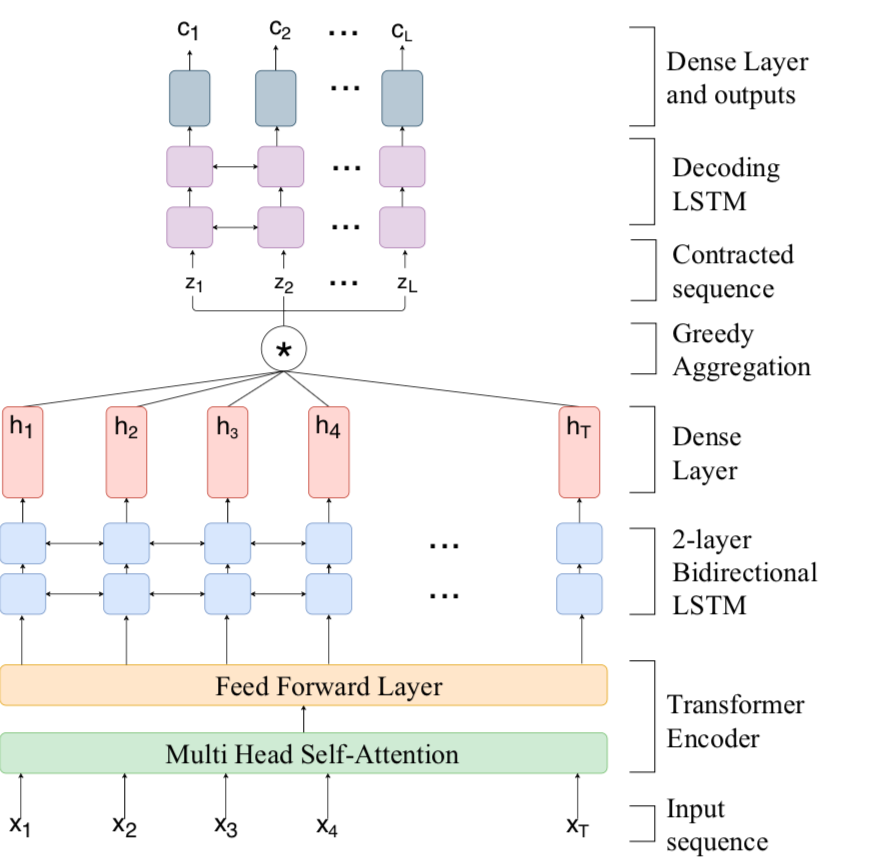# CTC Path Decoding

## Model

This module processes the input sequence of path coordinates to predict a sequence of English characters (which must be further transliterated into the Indic language) for the English-to-Indic decoding task and a sequence of Indic characters for the Indic-to-Indic decoding task. Consider an input sequence, {x1,x2,x3,…,xT } containing (x,y) path coordinates along with augmented features described in Section 3. As seen in Figure 2(a), the sequence is passed into a Transformer encoder consisting of a multi-head self-attention sub-layer and a position-wise feed forward neural network, followed by a 2-layer Bidirectional LSTM network (Schuster and Paliwal, 1997) to produce an encoded representation of the sequence. The encoded vector at each timestep is then passed through a fully connected layer with softmax activation to generate a sequence of vectors {h1,h2,h3,…,hT }; is the probability of the jth character at timestep i and |C|+1 is the number of characters including a blank character < b >. The model is trained using the CTC loss function which maximises the sum of probabilities of all frame-level alignments of the target sequence. Concretely, the CTC loss function maximises the probability:where x is the input sequence, y is the target sequence of length T andis the set of all frame-level alignments of y.Unlike conventional CTC-based models, we do not use greedy or beam-search path decoding to infer the character sequence directly from {h1,h2,h3,…,hT }. Instead, if all the vectors in a contiguous subsequence of length k (say, {hm,hm+1,hm+2,…,hm+k−1}) have the same most probable character (say, c), they are averaged to form a single vector:If c corresponding to an averaged vector is the blank character < b >, it is ignored and the remaining averaged vectors are concatenated into a new contracted sequence. We refer to this step as Greedy aggregation. This sequence is passed into a 2-layer Bidirectional LSTM which models the co-character dependence within the word, followed by a fully connected layer with softmax activation. The output gives the final probability distribution over the characters and the most probable character is chosen at each timestep.